Algebra is the metaphysics of arithmetic.

~ John Ray

First, as always, i hope everyone is safe, Second, as i mentioned in my last Snake_Byte [] let us do something a little more technical and scientific. For context, the catalyst for this was a surprising discussion that came from how current machine learning interviews are being conducted and how the basics of the distance between two vectors have been overlooked. So this is a basic example and in the following Snake_Byte [] i promise to get into something a little more say carnivore.

With that let us move to some linear algebra. For those that don’t know what linear algebra is, i will refer you to the best book on the subject, Professor Gilbert Strang’s Linear Algebra and its Applications.

i am biased here; however, i do believe the two most important areas of machine learning and data science are linear algebra and probability, with optimization techniques coming in a close third.

So dear reader, please bear with me here. We will review a little math; maybe for some, this will be new, and for those that already know this, you can rest your glass-balls.

We denote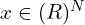be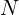-dimensional vectors taking real numbers as their entries. For example:where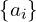are the indices respectively. In this case .

An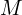-by-matrix is denoted as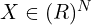. The transpose of a matrix is denoted as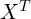. A matrix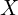can be viewed according to its columns and its rows: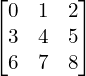where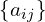are the row and column indices.

An array is a data structure in python programming that holds fix number of elements and these elements should be of the same data type. The main idea behind using an array of storing multiple elements of the same type. Most of the data structure makes use of an array to implement their algorithm. There is two important parts of the array:

• Element: Each item stored in the array is called an element.
• Index: Every element in the array has its own numerical value to identify the element.

Think of programming a loop, tuple, list,array,range or matrix:

from math import exp
v1 = [x, y] # list of variables
v2 = (-1, 2) # tuple of numbers
v3 = (x1, x2, x3) # tuple of variables

v4 = [exp(-i*0.1) for i in range(150)] #ye ole range loop

and check this out for a matrix:

import numpy as np
a = np.matrix('0 1:2 3')
print (a)
output: [[0 1]
[2 3]]


which folks is why we like the Snake Language. Really that is about it for vectors and matrices. The theory is where you get into proofs and derivations which can save you a ton of time on optimizations.

So now let’s double click on some things that will make you sound cool at the parties or meetups.

A vector can be multiplied by a number. This number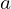is usually denoted as a scalar: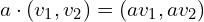Now given this one of the most fundamental aspects in all of machine-learning is the inner product, also called dot product, or scalar product, of two vectors, is a number. Most of all, machine learning algorithms have some form of a dot product somewhere within the depths of all the mathz. Nvidia GPUs are optimized for (you guessed it) dot products.

So how do we set this up? Multiplication of scalarand a vector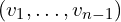yields: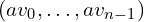Ok good so far.

The inner or dot product of two n-vectors is defined as: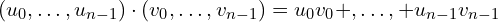which, if you are paying attention yields:

(1)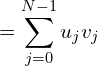Geometrically, the dot product of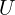and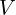equals the length oftimes the length oftimes the cosine of the angle between them: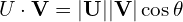ok so big deal huh? yea, but check this out in the Snake_Language:

# dot product of two vectors

# Importing numpy module
import numpy as np

# Taking two scalar values
a = 5
b = 7

# Calculating dot product using dot()
print(np.dot(a, b))
output: 35

hey now!

# Importing numpy module
import numpy as np

# Taking two 2D array
# For 2-D arrays it is the matrix product
a = [[2, 1], [0, 3]]
b = [[1, 1], [3, 2]]

# Calculating dot product using dot()
print(np.dot(a, b))
output:[[5 4]
[9 6]]

Mathematically speaking the inner product is a generalization of a dot product. As we said constructing a vector is done using the command np.array. Inside this command, one needs to enter the array. For a column vector, we write [,,], with an outer [], and three inner [] for each entry. If the vector is a row vector, the one can omit the inner []’s by just calling np.array([1, 2, 3]).

Given two column vectors x and y, the inner product is computed via np.dot(x.T,y), where np.dot is the command for inner product, and x.T returns the transpose of x. One can also call np.transpose(x), which is the same as x.T.

 # Python code to perform an inner product with transposition
import numpy as np
x = np.array([,,[-1]])
y = np.array([,,])
z = np.dot(np.transpose(x),y)
print (z) 

Yes, now dear read you now can impress your friends with your linear algebra and python prowess.

Note: In this case, the dot product is scale independent for actual purposes of real computation you must do something called a norm of a vector. i won’t go into the mechanics of this unless asked for further explanations on the mechanics of linear algebra. i will gladly go into pythonic examples if so asked and will be happy to write about said subject. Feel free to inquire in the comments below.

Unitl Then,

#iwishyouwater <- Nathan Florence with Kelly Slater at the Box. Watch.

tctjr.

Muzak to Blog By: INXS. i had forgotten how good of a band they were and the catalog. Michael Hutchinson, the lead singer, hung himself in a hotel room. Check out the song “By My Side”, “Dont Change” and “Never Tear Us Apart” and “To Look At You”. They weren’t afraid the take production chances.

Note: i resurrected some very old content from a previous site i owned i imported the older blogs. Some hilarious. Some sad. Some infuriating. i’m shining them up. Feel free to look back in time.